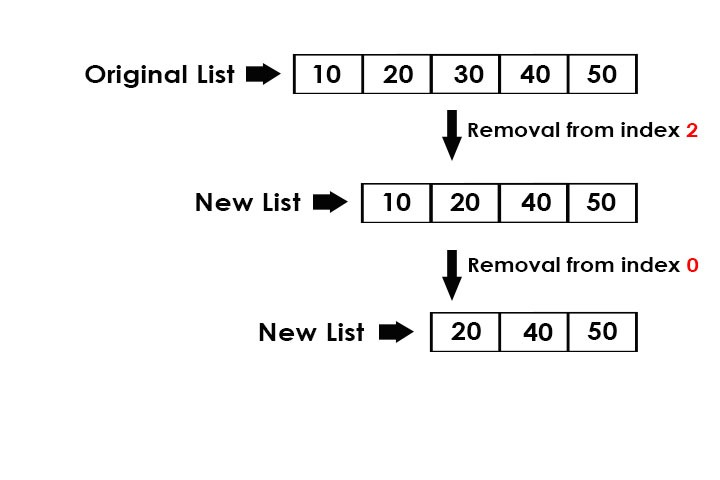GeeksforGeeks App
Open AppBrowser
Continue

# Python | Print list after removing element at given index

Given an index, remove the element at that index from the list and print the new list.Examples:

```Input : list = [10, 20, 30, 40, 50]
index = 2
Output : [10, 20, 40, 50]

Input : list = [10, 20, 40, 50]
index = 0
Output : [20, 40, 50] ```

## Recommended: Please try your approach on {IDE} first, before moving on to the solution.

Method 1: Traversal of list

Using traversal in the list, append all the index values except the given index to a new list and then print the new list. For this we will require a new list where we can append all the values except the given index value. Below is the Python3 implementation of the above approach

## Python3

 `# Python3 program to remove the index``# element from the list``# using traversal` `def` `remove(list1, pos):``    ``newlist ``=` `[]` `    ``# traverse in the list``    ``for` `x ``in` `range``(``len``(list1)):``        ` `        ``# if index not equal to pos``        ``if` `x !``=` `pos:``            ``newlist.append(list1[x])``    ``print``(``*``newlist) ` `# driver code``list1 ``=` `[``10``, ``20``, ``30``, ``40``, ``50``]``pos ``=` `2``remove(list1, pos)`

Output:

`10 20 40 50`

Method 2: pop()

pop() function helps us to pop the value at any desired position that is passed in the parameter, if nothing is passed in the parameter, then it removes the last index value. Below is the Python3 implementation of the above approach:

## Python3

 `# Python3 program to remove the index``# element from the list``# using pop()` `def` `remove(list1, pos):` `    ``# pop the element at index = pos``    ``list1.pop(pos)``    ``print``(``*``list1)``    ` `    ` `# driver code``list1 ``=` `[``10``, ``20``, ``30``, ``40``, ``50``]``pos ``=` `2``remove(list1, pos)`

Output:

`10 20 40 50`

Method 3: del function

del function can be used to remove any element at any given position. If -1 or -2 is given in the [] brackets, then it deletes the last and second last element respectively. Below is the Python3 implementation of the above approach:

## Python3

 `# Python3 program to remove the index element``# from the list using del` `def` `remove(list1, pos):` `    ``# delete the element at index = pos``    ``del` `list1[pos]``    ``print``(``*``list1)``    ` `    ` `# driver code``list1 ``=` `[``10``, ``20``, ``30``, ``40``, ``50``]``pos ``=` `2``remove(list1, pos)`

Output:

`10 20 40 50`

Approach#5: Using numpy.delete()

## note: install numpy module using command “pip install numpy”

Here’s the Python code for removing an element from a list at a given index using the numpy.delete() function, along with algorithm and time and space complexity analysis:

Algorithm:

Convert the input list to a NumPy array.
Use the numpy.delete() function to remove the element at the given index.
Convert the resulting NumPy array back to a Python list.
Print the new list.

## Python3

 `import` `numpy as np` `def` `remove(list1, pos):``    ``arr ``=` `np.array(list1)``    ``# Deleting element at given position using numpy.delete() function``    ``new_arr ``=` `np.delete(arr, pos)``    ``# Converting NumPy array back to list``    ``new_list ``=` `new_arr.tolist()``    ``# Printing updated list``    ``print``(``*``new_list)` `# driver code``list1 ``=` `[``10``, ``20``, ``30``, ``40``, ``50``]``pos ``=` `2``remove(list1, pos)`

Output:

10 20 40 50

Time complexity:
The numpy.delete() function has a time complexity of O(n), where n is the size of the input array. Converting the array to and from a list has a time complexity of O(n), where n is the size of the array. Therefore, the overall time complexity of the function is O(n).

Auxiliary Space:
The function uses extra space for the NumPy array, which has a space complexity of O(n), where n is the size of the input list. The conversion to and from a Python list also requires additional space, so the overall space complexity of the function is O(n).

My Personal Notes arrow_drop_up